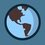Congratulations to one of the most brilliant young minds in the Brilliant community for passing the PMO Area Stage Qualifiers - @Sean Anderson Ty. I say that this is a great feat for there are many who had the chance but wasn't able to qualify (Yep, I'm talking about me) He also lives/studies in the same city as I am, so I am proud that maybe one of ours is going to be part of IMO.  As a gift, I give these Fibonacci problems (Who doesn't like proving?) 1) Let $m$ and $n$ be positive integers. Prove that, if $m$ is divisible by $n$, then, $f_{m}$ is divisible by $f_{n}$.  2) Let $m$ and $n$ be positive integers whose greatest common divisor is $d$. Prove that the greatest common divisor of the Fibonacci numbers $f_{m}$ and $f_{n}$ is the Fibonacci number $f_{d}$Note by Marc Vince Casimiro
6 years, 7 months ago

This discussion board is a place to discuss our Daily Challenges and the math and science related to those challenges. Explanations are more than just a solution — they should explain the steps and thinking strategies that you used to obtain the solution. Comments should further the discussion of math and science.

When posting on Brilliant:

• Use the emojis to react to an explanation, whether you're congratulating a job well done , or just really confused .
• Ask specific questions about the challenge or the steps in somebody's explanation. Well-posed questions can add a lot to the discussion, but posting "I don't understand!" doesn't help anyone.
• Try to contribute something new to the discussion, whether it is an extension, generalization or other idea related to the challenge.

MarkdownAppears as
*italics* or _italics_ italics
**bold** or __bold__ bold
- bulleted- list
• bulleted
• list
1. numbered2. list
1. numbered
2. list
Note: you must add a full line of space before and after lists for them to show up correctly
paragraph 1paragraph 2

paragraph 1

paragraph 2

[example link](https://brilliant.org)example link
> This is a quote
This is a quote
    # I indented these lines
# 4 spaces, and now they show
# up as a code block.

print "hello world"
# I indented these lines
# 4 spaces, and now they show
# up as a code block.

print "hello world"
MathAppears as
Remember to wrap math in $$ ... $$ or $ ... $ to ensure proper formatting.
2 \times 3 $2 \times 3$
2^{34} $2^{34}$
a_{i-1} $a_{i-1}$
\frac{2}{3} $\frac{2}{3}$
\sqrt{2} $\sqrt{2}$
\sum_{i=1}^3 $\sum_{i=1}^3$
\sin \theta $\sin \theta$
\boxed{123} $\boxed{123}$

Sort by:

- 6 years, 7 months ago

@Sean Ty Congrats!

Staff - 6 years, 7 months ago